# 十三、对象跟踪

• 如何使用帧差异
• 如何使用色彩空间跟踪有色对象
• 如何构建交互式对象跟踪器
• 如何构建特征跟踪器
• 如何建立视频监控系统

# 帧的差异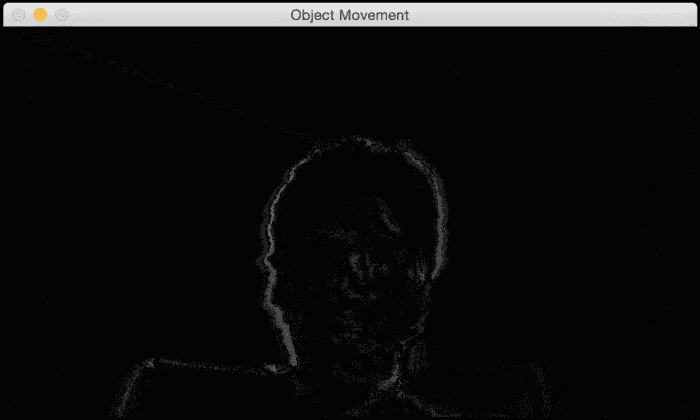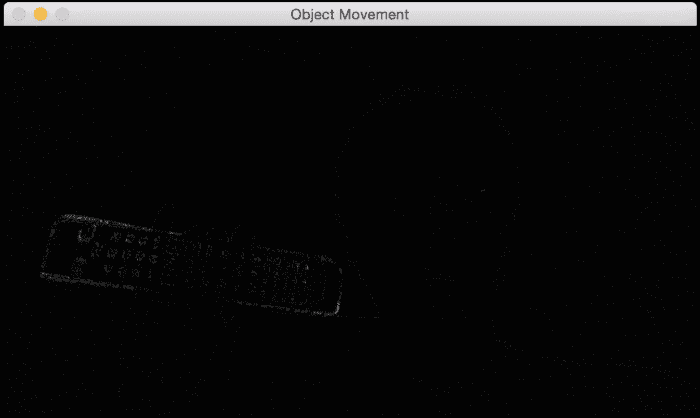import cv2

# Compute the frame difference
def frame_diff(prev_frame, cur_frame, next_frame):
# Absolute difference between current frame and next frame
diff_frames1 = cv2.absdiff(next_frame, cur_frame)

# Absolute difference between current frame and # previous frame
diff_frames2 = cv2.absdiff(cur_frame, prev_frame)

# Return the result of bitwise 'AND' between the # above two resultant images
return cv2.bitwise_and(diff_frames1, diff_frames2)

# Capture the frame from webcam
def get_frame(cap):
# Capture the frame

# Resize the image
frame = cv2.resize(frame, None, fx=scaling_factor,
fy=scaling_factor, interpolation=cv2.INTER_AREA)

# Return the grayscale image
return cv2.cvtColor(frame, cv2.COLOR_RGB2GRAY)

if __name__=='__main__':
cap = cv2.VideoCapture(0)
scaling_factor = 0.5

prev_frame = get_frame(cap)
cur_frame = get_frame(cap)
next_frame = get_frame(cap)

# Iterate until the user presses the ESC key
while True:
# Display the result of frame differencing
cv2.imshow("Object Movement", frame_diff(prev_frame, cur_frame, next_frame))

# Update the variables
prev_frame = cur_frame
cur_frame = next_frame
next_frame = get_frame(cap)

# Check if the user pressed ESC
key = cv2.waitKey(10)
if key == 27:
break

cv2.destroyAllWindows()


# 基于色彩空间的跟踪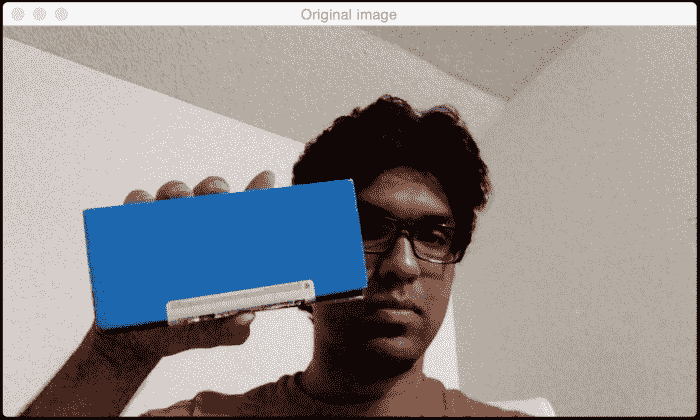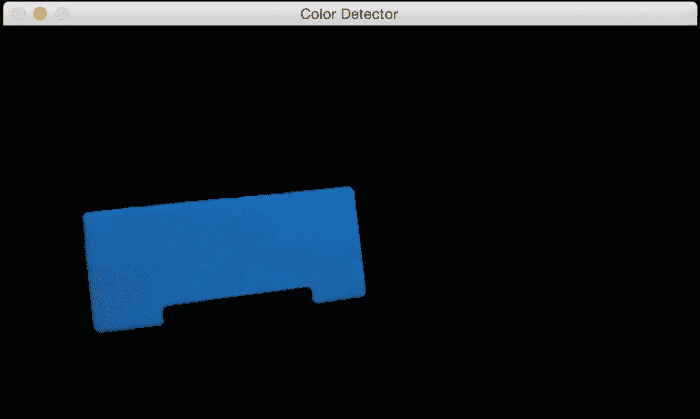import cv2
import numpy as np

# Capture the input frame from webcam
def get_frame(cap, scaling_factor):
# Capture the frame from video capture object

# Resize the input frame
frame = cv2.resize(frame, None, fx=scaling_factor,
fy=scaling_factor, interpolation=cv2.INTER_AREA)

return frame

if __name__=='__main__':
cap = cv2.VideoCapture(0)
scaling_factor = 0.5

# Iterate until the user presses ESC key
while True:
frame = get_frame(cap, scaling_factor)

# Convert the HSV colorspace
hsv = cv2.cvtColor(frame, cv2.COLOR_BGR2HSV)

# Define 'blue' range in HSV colorspace
lower = np.array([60,100,100])
upper = np.array([180,255,255])

# Threshold the HSV image to get only blue color

# Bitwise-AND mask and original image
res = cv2.medianBlur(res, 5)

cv2.imshow('Original image', frame)
cv2.imshow('Color Detector', res)

# Check if the user pressed ESC key
c = cv2.waitKey(5)
if c == 27:
break

cv2.destroyAllWindows()


# 构建交互式对象跟踪器

Meanshift 的概念实际上很简单。 假设我们选择了一个兴趣区域，并且希望我们的对象跟踪器跟踪该对象。 在该区域中，我们基于颜色直方图选择一堆点并计算质心。 如果质心位于该区域的中心，则说明该对象没有移动。 但是，如果质心不在此区域的中心，那么我们知道对象正在朝某个方向移动。 重心的移动控制对象移动的方向。 因此，我们将边界框移动到新位置，以便新质心成为此边界框的中心。 因此，此算法称为均值移位，因为均值（即质心）正在移动。 这样，我们就可以使用对象的当前位置进行更新。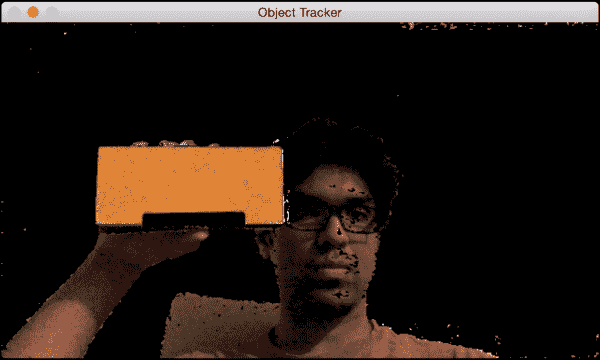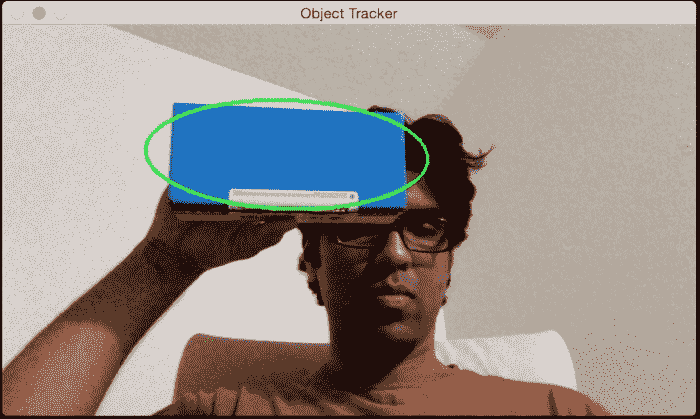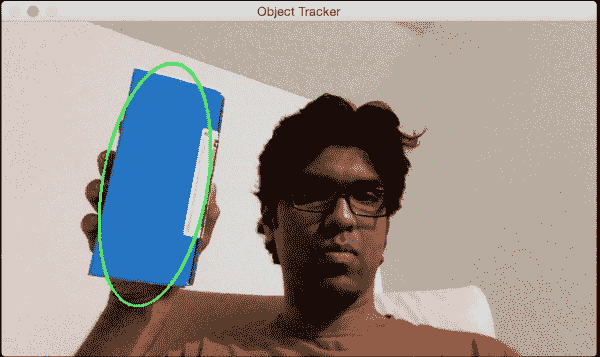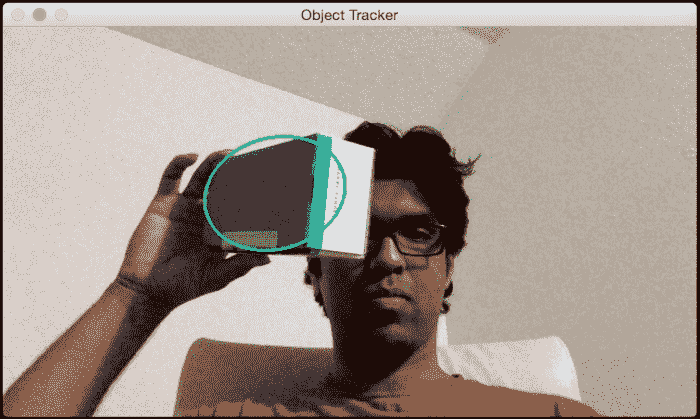import sys

import cv2
import numpy as np

class ObjectTracker(object):
def __init__(self):
# Initialize the video capture object
# 0 -> indicates that frame should be captured
# from webcam
self.cap = cv2.VideoCapture(0)

# Capture the frame from the webcam

# Downsampling factor for the input frame
self.scaling_factor = 0.5
self.frame = cv2.resize(self.frame, None, fx=self.scaling_factor,
fy=self.scaling_factor, interpolation=cv2.INTER_AREA)

cv2.namedWindow('Object Tracker')
cv2.setMouseCallback('Object Tracker', self.mouse_event)

self.selection = None
self.drag_start = None
self.tracking_state = 0

# Method to track mouse events
def mouse_event(self, event, x, y, flags, param):
x, y = np.int16([x, y])

# Detecting the mouse button down event
if event == cv2.EVENT_LBUTTONDOWN:
self.drag_start = (x, y)
self.tracking_state = 0

if self.drag_start:
if flags & cv2.EVENT_FLAG_LBUTTON:
h, w = self.frame.shape[:2]
xo, yo = self.drag_start
x0, y0 = np.maximum(0, np.minimum([xo, yo], [x, y]))
x1, y1 = np.minimum([w, h], np.maximum([xo, yo], [x, y]))
self.selection = None

if x1-x0 > 0 and y1-y0 > 0:
self.selection = (x0, y0, x1, y1)

else:
self.drag_start = None
if self.selection is not None:
self.tracking_state = 1

# Method to start tracking the object
def start_tracking(self):
# Iterate until the user presses the Esc key
while True:
# Capture the frame from webcam
# Resize the input frame
self.frame = cv2.resize(self.frame, None, fx=self.scaling_factor,
fy=self.scaling_factor, interpolation=cv2.INTER_AREA)

vis = self.frame.copy()

# Convert to HSV colorspace
hsv = cv2.cvtColor(self.frame, cv2.COLOR_BGR2HSV)

# Create the mask based on predefined thresholds.
mask = cv2.inRange(hsv, np.array((0., 60., 32.)),
np.array((180., 255., 255.)))

if self.selection:
x0, y0, x1, y1 = self.selection
self.track_window = (x0, y0, x1-x0, y1-y0)
hsv_roi = hsv[y0:y1, x0:x1]

# Compute the histogram
hist = cv2.calcHist( [hsv_roi], , mask_roi, , [0, 180] )

# Normalize and reshape the histogram
cv2.normalize(hist, hist, 0, 255, cv2.NORM_MINMAX);
self.hist = hist.reshape(-1)

vis_roi = vis[y0:y1, x0:x1]
cv2.bitwise_not(vis_roi, vis_roi)

if self.tracking_state == 1:
self.selection = None

# Compute the histogram back projection
prob = cv2.calcBackProject([hsv], , self.hist, [0, 180], 1)

term_crit = ( cv2.TERM_CRITERIA_EPS | cv2.TERM_CRITERIA_COUNT, 10, 1 )

# Apply CAMShift on 'prob'
track_box, self.track_window = cv2.CamShift(prob, self.track_window, term_crit)

# Draw an ellipse around the object
cv2.ellipse(vis, track_box, (0, 255, 0), 2)

cv2.imshow('Object Tracker', vis)

c = cv2.waitKey(5)
if c == 27:
break

cv2.destroyAllWindows()

if __name__ == '__main__':
ObjectTracker().start_tracking()


# 基于特征的跟踪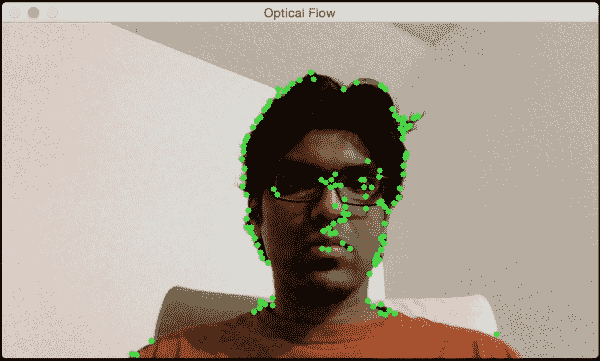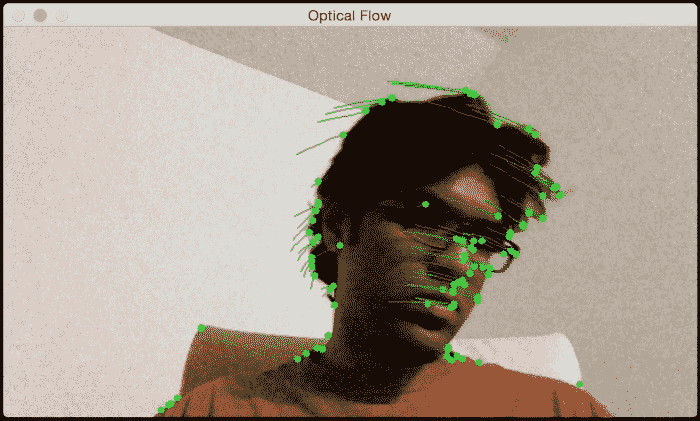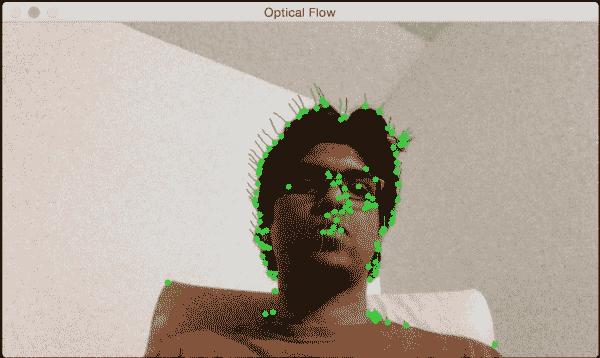import cv2
import numpy as np

def start_tracking():
# Capture the input frame
cap = cv2.VideoCapture(0)

# Downsampling factor for the image
scaling_factor = 0.5

# Number of frames to keep in the buffer when you
# are tracking. If you increase this number,
# feature points will have more "inertia"
num_frames_to_track = 5

# Skip every 'n' frames. This is just to increase the speed.
num_frames_jump = 2

tracking_paths = []
frame_index = 0

# 'winSize' refers to the size of each patch. These patches
# are the smallest blocks on which we operate and track
# here: http://goo.gl/ulwqLk
tracking_params = dict(winSize  = (11, 11), maxLevel = 2,
criteria = (cv2.TERM_CRITERIA_EPS | cv2.TERM_CRITERIA_COUNT, 10, 0.03))

# Iterate until the user presses the ESC key
while True:

# downsample the input frame
frame = cv2.resize(frame, None, fx=scaling_factor,
fy=scaling_factor, interpolation=cv2.INTER_AREA)

frame_gray = cv2.cvtColor(frame, cv2.COLOR_BGR2GRAY)
output_img = frame.copy()

if len(tracking_paths) > 0:
prev_img, current_img = prev_gray, frame_gray
feature_points_0 = np.float32([tp[-1] for tp in tracking_paths]).reshape(-1, 1, 2)

# Compute feature points using optical flow. You can
# parameters here: http://goo.gl/t6P4SE
feature_points_1, _, _ = cv2.calcOpticalFlowPyrLK(prev_img, current_img, feature_points_0,
None, **tracking_params)
feature_points_0_rev, _, _ = cv2.calcOpticalFlowPyrLK(current_img, prev_img, feature_points_1,
None, **tracking_params)

# Compute the difference of the feature points
diff_feature_points = abs(feature_points_0- feature_points_0_rev).reshape(-1, 2).max(-1)

# threshold and keep the good points
good_points = diff_feature_points < 1

new_tracking_paths = []

for tp, (x, y), good_points_flag in zip(tracking_paths,
feature_points_1.reshape(-1, 2), good_points):
if not good_points_flag:
continue

tp.append((x, y))

# Using the queue structure i.e. first in,
# first out
if len(tp) > num_frames_to_track:
del tp

new_tracking_paths.append(tp)

# draw green circles on top of the output image
cv2.circle(output_img, (x, y), 3, (0, 255, 0), -1)

tracking_paths = new_tracking_paths

# draw green lines on top of the output image
cv2.polylines(output_img, [np.int32(tp) for tp in tracking_paths], False, (0, 150, 0))

# 'if' condition to skip every 'n'th frame
if not frame_index % num_frames_jump:
for x, y in [np.int32(tp[-1]) for tp in tracking_paths]:
cv2.circle(mask, (x, y), 6, 0, -1)

# about the parameters here: http://goo.gl/BI2Kml
feature_points = cv2.goodFeaturesToTrack(frame_gray,
minDistance = 7, blockSize = 7)

if feature_points is not None:
for x, y in np.float32(feature_points).reshape (-1, 2):
tracking_paths.append([(x, y)])

frame_index += 1
prev_gray = frame_gray

cv2.imshow('Optical Flow', output_img)

# Check if the user pressed the ESC key
c = cv2.waitKey(1)
if c == 27:
break

if __name__ == '__main__':
start_tracking()
cv2.destroyAllWindows()


# 背景减法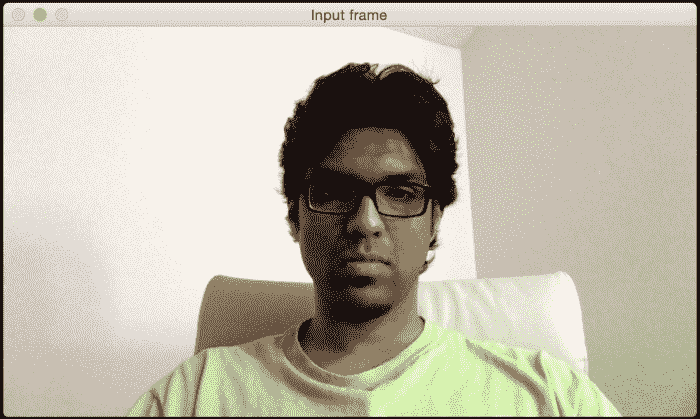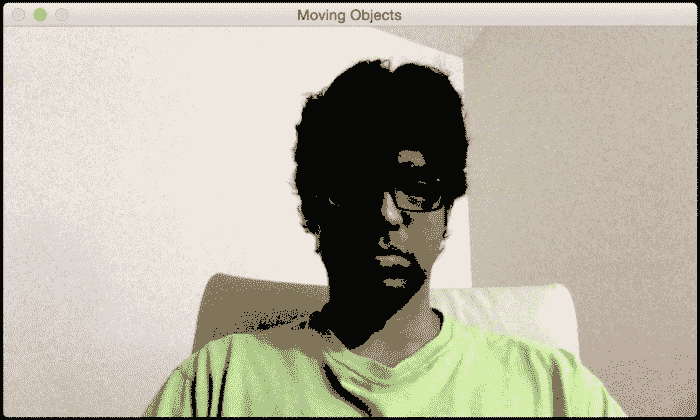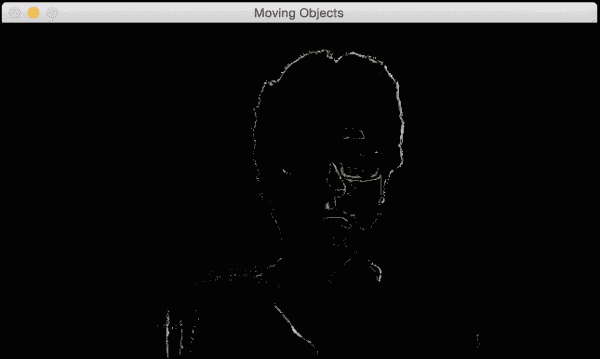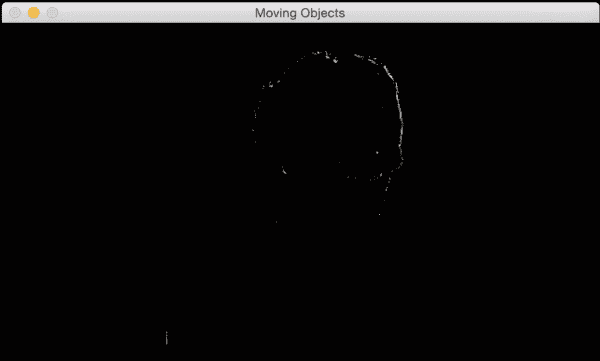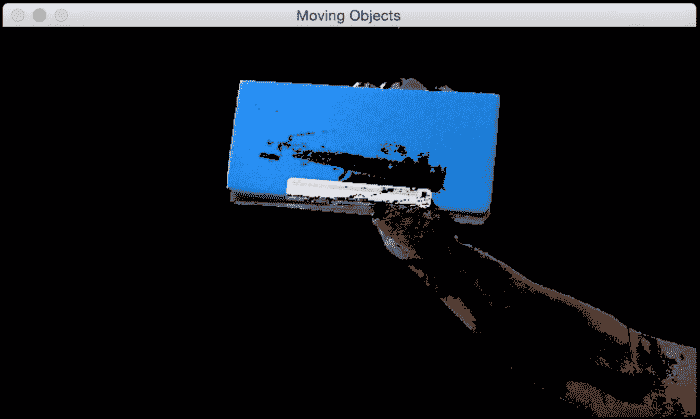import cv2
import numpy as np

# Capture the input frame
def get_frame(cap, scaling_factor=0.5):

# Resize the frame
frame = cv2.resize(frame, None, fx=scaling_factor,
fy=scaling_factor, interpolation=cv2.INTER_AREA)

return frame

if __name__=='__main__':
# Initialize the video capture object
cap = cv2.VideoCapture(0)

# Create the background subtractor object
bgSubtractor = cv2.BackgroundSubtractorMOG()

# This factor controls the learning rate of the algorithm.
# The learning rate refers to the rate at which your model
# will learn about the background. Higher value for
# 'history' indicates a slower learning rate. You
# can play with this parameter to see how it affects
# the output.
history = 100

# Iterate until the user presses the ESC key
while True:
frame = get_frame(cap, 0.5)

# Apply the background subtraction model to the # input frame

# Convert from grayscale to 3-channel RGB

cv2.imshow('Input frame', frame)

# Check if the user pressed the ESC key
c = cv2.waitKey(10)
if c == 27:
break

cap.release()
cv2.destroyAllWindows()